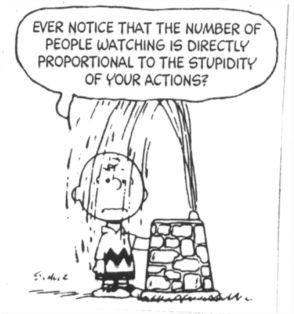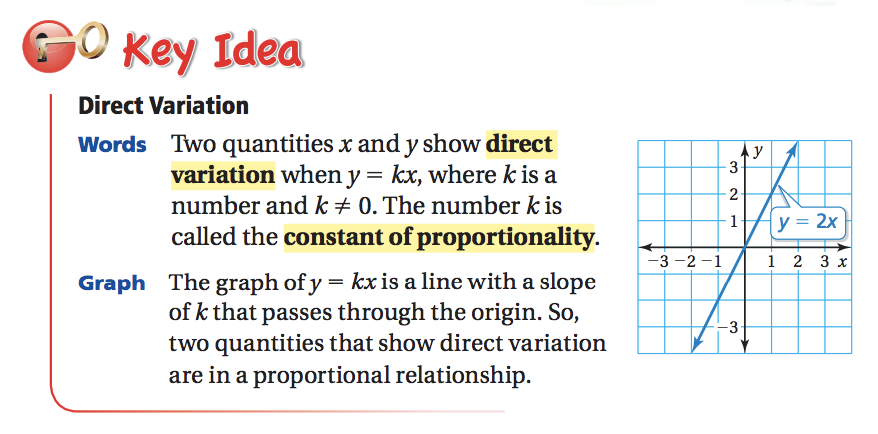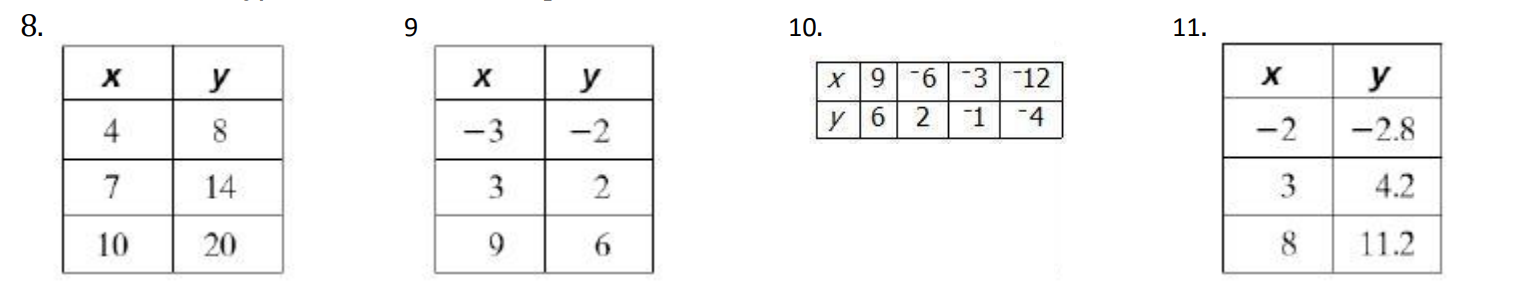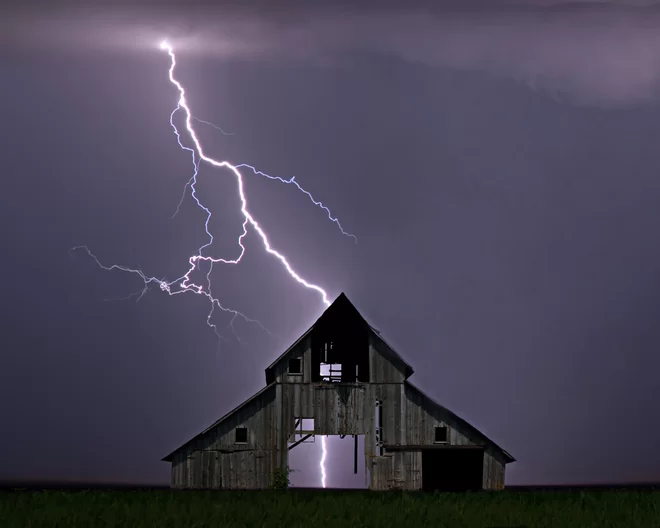Direct Variation and Chapter 5 Review
starstarstarstarstarstarstarstarstarstar
by Felix Gabathuler
| 36 Questions1
1
What is the value of the expression below?

2
1

3
1
The school store sells 4 pencils for \$0.80. What is the unit cost of a pencil?
4
1
Solve and plot the inequality -2x + 1 < 5 on a number line.
5
1
What is the missing number (x) that would make the following two ratios proportional?

6
1
What is the missing number (x) that would make the following two ratios proportional?

7
1
True of false: the table below representing x and y are in a proportional relationship.

True
False
8
1
Plot the lines from the table below onto a coordinate plane. Connect those lines with a coordinate plane. In addition, plot a point for coordinate (0, y) and (4, y). What would the y values be for those coordinates? You should plot five points in total.
9
1
What is the slope of the line that is being represented by the graph below?

10
1
What would be the cost of 7 avocados?11
1
Use the table to write a proportion.

12
1

A school requires 2 computers for every 5 students. Write a proportion that gives the number c of computers needed for 145 students.
13
1

A school requires 2 computers for every 5 students. Write a proportion that gives the number c of computers needed for 145 students. Now solve for c.
14
1
In an orchestra, the ratio of trombones to violas is 1 to 3.
There are 9 violas. Write a proportion that gives the number t of trombones in the orchestra (only use #s in your answer).
15
1
In an orchestra, the ratio of trombones to violas is 1 to 3.
There are 9 violas. How many trombones are in the orchestra?
16
1
Find the slope of the line.

17
1
Without using a graph, find the slope of the line that passes through the following points:

18
1
What is the slope of a line that passes through the points (2, 0) and (5, 0)?19
1
After watching the video and reviewing the definitions from the book, write down the important information in your notebook. Next, write how you would explain the concept of direct variation to your mom. How does it relate to slope, ratios, rates, and proportions? Write at least 3 sentences detailing your explanation.
20
1
If you had to sum up the concept of direct variation in one word, what word would that be...?
21
1
True/False: x and y show direct variation

True
False
22
1
Explain your reasoning for whether the ratio table below represents direction variation. Use specific examples in your argument.

Watch the next few videos to get a better feel of direct variation and how it applies to real world situations. If you're feeling extremely confident about it, move on. If not, just watch. They're not very long.
23
1
Now let's determine if a ratio table represents direct variation. Remember, a ratio table is just a collection of coordinate (x,y) values that make up a line on a graph. But first, what are some defining characteristics of a line that represents direct variation?
We can tell if a table represents direct variation if the slope is the same between all the points. You can also tell by choosing two points and making them proportional to each other. If they reduce to the same number, they represent direct variation! Try the following to test your skills!24
25
26
27
24
1
Write yes or no for whether this equation represents direct variation. If the answer is yes, find the constant of variation (slope). Write or answer like this: yes,k=3 or no
25
1
Write yes or no for whether this equation represents direct variation. If the answer is yes, find the constant of variation (slope). Write or answer like this: yes,k=3 or no
26
1
Write yes or no for whether this equation represents direct variation. If the answer is yes, find the constant of variation (slope). Write or answer like this: yes,k=3 or no
27
1
Write yes or no for whether this equation represents direct variation. If the answer is yes, find the constant of variation (slope). Write or answer like this: yes,k=3 or no.
28
1
On the graph below, draw a line that represents direct variation that passes through the point (2,3)
29
1
Tell whether x and y show direct variation. How can you tell? Explain how you know.30
1
Your distance (d) from lightning varies directly with the time (t) it takes you to hear thunder. If you hear thunder 10 seconds after you see the lightening, you are about 2 miles from the lightning.

Write a direct variation equation for the relationship between time (t) and distance (d).
31
1
Use the equation from above to determine how long it would take for the sound of thunder to travel 6 miles. Only write the number, no units.32
1
A recipe for 3 dozen corn muffins calls for 2 cups of flour. The number of muffins (m) varies directly with the amount of flour (f) you use.

Write a direct variation equation for the relationship between the number of cups of flour (f) and how many muffins (m) it would make.
33
1
Using the equation from above, determine how many cups of flour are needed to make 10 dozen muffins. Write your answer as a reduced improper fraction. Do not include units.34
1
Using the digits 1 to 9, at most one time each, place a digit in each box to make a true statement.
35
1
Use the whole numbers 1 through 9 only one time each to find the largest (or smallest) possible values for the sum of x and y.
36
1
Using the numbers 0 through 9, at most one time each, fill in the boxes to create a correct sentence: